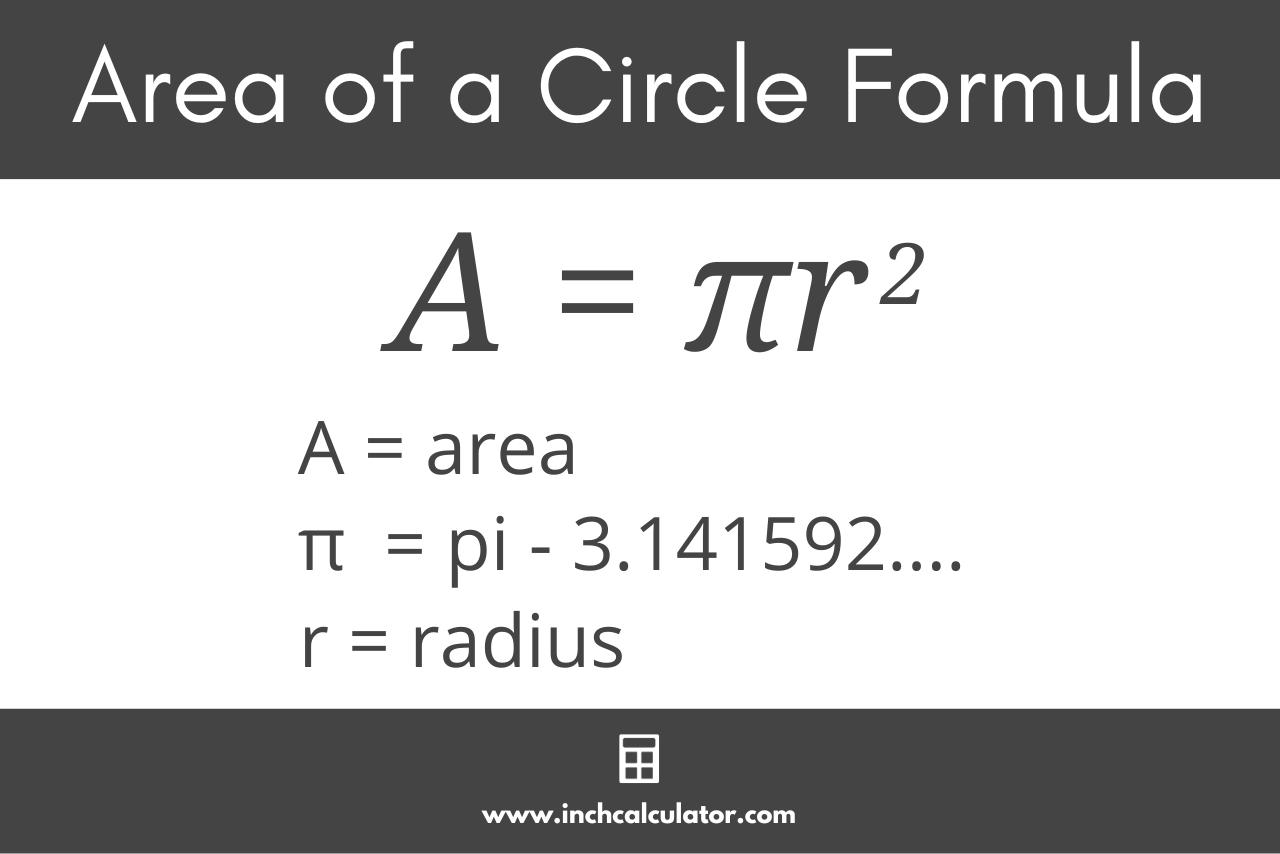# Area of a Circle Calculator

Calculate the area of a circle using the radius, diameter, or circumference with the circle area calculator.

## How to Calculate the Area of a Circle

A circle is a round, two-dimensional shape with no corners or edges where each point along its edge is equidistant from the center. The area of a circle is the amount of space the shape occupies.

Think of the area of a circle as the region of a two-dimensional plane it occupies or encloses.

There are a few ways to find the area of a circle.

The first way to calculate area is to use the circle area calculator above. The second way is to use a circle area formula.

### Circle Area Formulas

To calculate the area of a circle, you’ll need its radius, diameter, or circumference.

Then, use one of the following formulas.

The radius of a circle is the length of a straight line from the center of the circle to the outer edge. The most commonly used formula to find the area given the radius of a circle is:

A = π × r²

Thus, the area of a circle A is equal to pi times the radius r squared.#### Find Area Using Diameter

The diameter is the length of a straight line from one edge of the circle to the opposite edge that passes through its center. If the line does not pass through the center of the circle, it is called a chord, not the diameter. The diameter is twice the length of the radius.

The formula to find the area of a circle given its diameter is:

A = π × (d / 2

The area A is equal to pi times the diameter d divided by 2, squared.

#### Find Area Using Circumference

The circumference of a circle is the length of the outer edge around its perimeter. The formula to find the area of a circle given its circumference is:

A = π × (C /

The area A is equal to pi times the circumference C divided by 2 times pi, squared.

Give our circle calculator a try and solve a circle’s dimensions, including the radius, diameter, and circumference.

### What number does π represent?

π, called pi, is a natural number occurring in nature that is equal to approximately 3.14159265. Fun fact, pi is an irrational number, meaning its decimal has an infinite number of digits.

### Why is the area of a circle equal to πr²?

If you divide a circle into many equivalent slices, like a pizza, and fit the slices together like a zipper, the shape resembles a parallelogram. The area of a parallelogram is equal to its height times its base.

In this case, the height of the parallelogram is equal to the circle’s radius, and the base, when measured, turns out to be approximately 3.14 times the radius. Therefore, its area is equal to 3.14 times r².

If you divide the circle into more and more slices, the 3.14 value gets closer and closer to the value of pi. Thus, the area of a circle is equal to πr².

### How do you solve πr²?

Since pi is an irrational number, we must use an approximation for it when calculating the area of a circle. Normally we use 3.14 as the approximation, or 3.14159265 if you want to be more accurate.

Therefore, as an example, if the radius of a circle is 2, then its area, A, is

A = πr² = 3.14 × 2² = 3.14 × 4 = 12.56

### Why is pi used to find the area of a circle?

Pi is used to find the area of a circle because it is simply a naturally occurring number in nature that humans discovered when first deriving the formula to calculate the area of a circle.

They found the area was equal to some number times the radius squared, but as they used better and better approximations for the area, they discovered that number to be pi!

### Is area the same as the circumference?

No. The area of a circle is the space inside the circle, while the circumference is the length around the circle. The units of circumference are in length, while the area is in length squared.